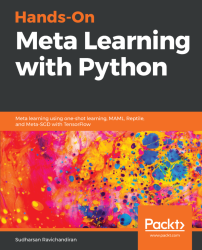•#### Hands-On Meta Learning with Python#### Overview of this book

Meta learning is an exciting research trend in machine learning, which enables a model to understand the learning process. Unlike other ML paradigms, with meta learning you can learn from small datasets faster. Hands-On Meta Learning with Python starts by explaining the fundamentals of meta learning and helps you understand the concept of learning to learn. You will delve into various one-shot learning algorithms, like siamese, prototypical, relation and memory-augmented networks by implementing them in TensorFlow and Keras. As you make your way through the book, you will dive into state-of-the-art meta learning algorithms such as MAML, Reptile, and CAML. You will then explore how to learn quickly with Meta-SGD and discover how you can perform unsupervised learning using meta learning with CACTUs. In the concluding chapters, you will work through recent trends in meta learning such as adversarial meta learning, task agnostic meta learning, and meta imitation learning. By the end of this book, you will be familiar with state-of-the-art meta learning algorithms and able to enable human-like cognition for your machine learning models.
Title PageDedicationContributorsPrefaceFree Chapter
Introduction to Meta LearningFace and Audio Recognition Using Siamese NetworksPrototypical Networks and Their VariantsRelation and Matching Networks Using TensorFlowMemory-Augmented Neural NetworksMAML and Its VariantsMeta-SGD and ReptileGradient Agreement as an Optimization ObjectiveAssessmentsOther Books You May EnjoyIndex## Reptile

The Reptile algorithm has been proposed as an improvement to MAML by OpenAI. It's simple and easier to implement. We know that, in MAML, we calculate second order derivatives—that is, the gradient of gradients. But computationally, this isn't an efficient task. So, OpenAI came up with an improvement over MAML called Reptile. The algorithm of Reptile is very simple. Sample some n number of tasks and run Stochastic Gradient Descent (SGD) for fewer iterations on each of the sampled tasks and then update our model parameter in a direction that's common to all of the tasks. Since we're performing SGD for fewer iterations on each task, it indirectly implies we're calculating the second order derivative over the loss. Unlike MAML, it's computationally effective as we're not calculating the second order derivative directly nor unrolling the computational graph, and so it is easier to implement.

Let's say we sampled two tasks,and, from the task distribution and we randomly initialize the...